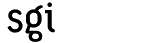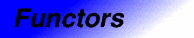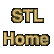# mem_fun1_t<Result, X, Arg>Categories: functors, adaptors Component type: type

### Description

Mem_fun1_t is an adaptor for member functions. If X is some class with a member function Result X::f(Arg) (that is, a member function that takes one argument of type Arg and that returns a value of type Result ), then a mem_fun1_t<Result, X, Arg> is a function object adaptor that makes it possible to call f as if it were an ordinary function instead of a member function.

Mem_fun1_t<Result, X, Arg>'s constructor takes a pointer to one of X's member functions. Then, like all function objects, mem_fun1_t has an operator() that allows the mem_fun1_t to be invoked with ordinary function call syntax. In this case, mem_fun1_t's operator() takes two arguments; the first is of type X* and the second is of type Arg.

If F is a mem_fun1_t that was constructed to use the member function X::f, and if x is a pointer of type X* and a is a value of type Arg, then the expression F(x, a) is equivalent to the expression x->f(a). The difference is simply that F can be passed to STL algorithms whose arguments must be function objects.

Mem_fun1_t is one of a family of member function adaptors. These adaptors are useful if you want to combine generic programming with inheritance and polymorphism, since, in C++, polymorphism involves calling member functions through pointers or references.

As with many other adaptors, it is usually inconvenient to use mem_fun1_t's constructor directly. It is usually better to use the helper function mem_fun  instead.

### Example

```struct Operation {
virtual double eval(double) = 0;
};

struct Square : public Operation {
double eval(double x) { return x * x; }
};

struct Negate : public Operation {
double eval(double x) { return -x; }
};

int main() {
vector<Operation*> operations;
vector<double> operands;

operations.push_back(new Square);
operations.push_back(new Square);
operations.push_back(new Negate);
operations.push_back(new Negate);
operations.push_back(new Square);

operands.push_back(1);
operands.push_back(2);
operands.push_back(3);
operands.push_back(4);
operands.push_back(5);

transform(operations.begin(), operations.end(),
operands.begin(),
ostream_iterator<double>(cout, "\n"),
mem_fun(Operation::eval));
}
```

### Definition

Defined in the standard header functional, and in the nonstandard backward-compatibility header function.h.

### Template parameters

Parameter Description Default
Result The member function's return type.
X The class whose member function the mem_fun1_t invokes.
Arg The member function's argument type.

### Type requirements

• X has at least one member function that takes a single argument of type Arg and that returns a value of type Result. 

### Public base classes

binary_function<X*, Arg, Result>

### Members

Member Where defined Description
first_argument_type Adaptable Binary Function The type of the first argument: X*
second_argument_type Adaptable Binary Function The type of the second argument: Arg
result_type Adaptable Binary Function The type of the result: Result
```Result operator()(X* x, Arg a) const
```
Binary Function Function call operator. Invokes x->f(a), where f is the member function that was passed to the constructor.
```explicit mem_fun1_t(Result (X::*f)(Arg))
```
mem_fun1_t See below.
```template <class Result, class X, class Arg>
mem_fun1_t<Result, X, Arg>
mem_fun(Result (X::*f)(Arg)); 
```
mem_fun1_t See below.

### New members

These members are not defined in the Adaptable Binary Function requirements, but are specific to mem_fun1_t.
Member Description
```explicit mem_fun1_t(Result (X::*f)(Arg))
```
The constructor. Creates a mem_fun1_t that calls the member function f.
```template <class Result, class X, class Arg>
mem_fun1_t<Result, X, Arg>
mem_fun(Result (X::*f)(Arg)); 
```
If f is of type Result (X::*)(Arg) then mem_fun(f) is the same as mem_fun1_t<Result, X, Arg>(f), but is more convenient. This is a global function, not a member function.

### Notes

 The type Result is permitted to be void. That is, this adaptor may be used for functions that return no value. However, this presents implementation difficulties. According to the draft C++ standard, it is possible to return from a void function by writing return void instead of just return. At present, however (early 1998), very few compilers support that feature. As a substitute, then, mem_fun1_t uses partial specialization to support void member functions. If your compiler has not implemented partial specialization, then you will not be able to use mem_fun1_t with member functions whose return type is void.

 This helper function was called mem_fun1 in drafts of the C++ standard, but it is called mem_fun in the final standard. This implementation provides both versions for backward compatibility, but mem_fun1 will be removed in a future release.